numpy.random.RandomState.poisson¶

RandomState.poisson(lam=1.0, size=None)

Draw samples from a Poisson distribution.

The Poisson distribution is the limit of the Binomial distribution for large N.

Parameters : lam : float Expectation of interval, should be >= 0. size : int or tuple of ints, optional Output shape. If the given shape is, e.g., (m, n, k), then m * n * k samples are drawn.

Notes

The Poisson distribution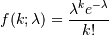For events with an expected separation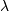the Poisson distribution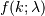describes the probability of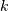events occurring within the observed interval.

Because the output is limited to the range of the C long type, a ValueError is raised when lam is within 10 sigma of the maximum representable value.

References

 [R161] Weisstein, Eric W. “Poisson Distribution.” From MathWorld–A Wolfram Web Resource. http://mathworld.wolfram.com/PoissonDistribution.html
 [R162] Wikipedia, “Poisson distribution”, http://en.wikipedia.org/wiki/Poisson_distribution

Examples

Draw samples from the distribution:

>>> import numpy as np
>>> s = np.random.poisson(5, 10000)

Display histogram of the sample:

>>> import matplotlib.pyplot as plt
>>> count, bins, ignored = plt.hist(s, 14, normed=True)
>>> plt.show()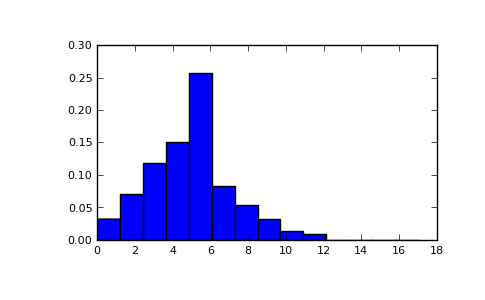Previous topic

numpy.random.RandomState.permutation

Next topic

numpy.random.RandomState.power